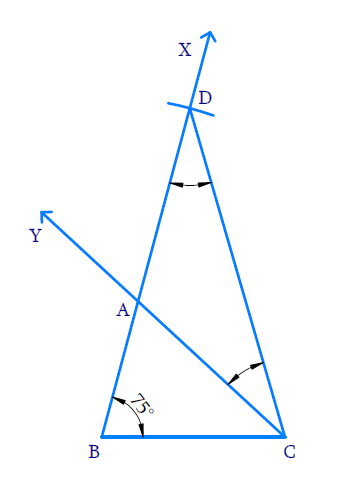In the verge of coronavirus pandemic, we are providing FREE access to our entire Online Curriculum to ensure Learning Doesn't STOP!

# Ex.11.2 Q1 Constructions Solution - NCERT Maths Class 9

Go back to  'Ex.11.2'

## Question

Construct a triangle $$ABC$$ in which $$BC =$$ $$7\;\rm{cm}$$ , $$\angle B$$ = $$75°$$ and \begin{align}\mathrm{AB}+\mathrm{AC}=13 \mathrm{cm}\end{align}

Video Solution
Constructions
Ex 11.2 | Question 1

## Text Solution

Steps of Construction:(i) Draw base  $$BC =7\;\rm{cm}$$ and at point $$B$$ make an angle of $$75^\circ$$ using protractor.

(ii) With $$B$$ as center and radius equal to $$13\;\rm{cm}$$, draw an arc to intersect ray $$BX$$ at $$D$$.

(iii) Join $$DC$$

(iv) Measure $$\angle \mathrm{D}$$  and make  $$\angle \mathrm{ACD}=\angle \mathrm{D}$$

(v) Let $$CY$$ intersect $$BD$$ at $$A$$

(vi) $$ABC$$ is the required triangle

Learn from the best math teachers and top your exams

• Live one on one classroom and doubt clearing
• Practice worksheets in and after class for conceptual clarity
• Personalized curriculum to keep up with school